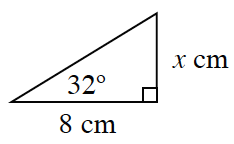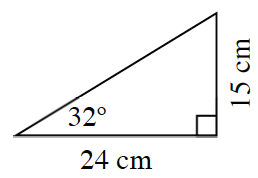### Home > CC3MN > Chapter 9 > Lesson 9.1.1 > Problem9-6

9-6.

Consider the triangles at right:

1. Are the two triangles at right similar? How do you know?

Two triangles are similar if they have the same angle measures. Because of the Angle Sum Theorem, you only need to know two angles are congruent to know that two triangles are similar.

2. Find the value of $x$. Show your strategy.

Try finding the scale factor between the two corresponding sides that are given and solve for $x$.

Another way to solve for $x$ is to create a proportion and cross multiply. One possible proportion is shown at right.

$\frac{24}{8}=\frac{15}{\it x}$

$x = 5$ cm

3. What is the scale factor ($\frac{\text{big}}{\text{small}}$)?

What is the scale factor between the larger triangle and the smaller triangle? Find the ratio between corresponding sides.

$3$

4. Find the area of each triangle.

Use the formula for area of a triangle:

$\frac{1}{2}(\text{base})(\text{height})$